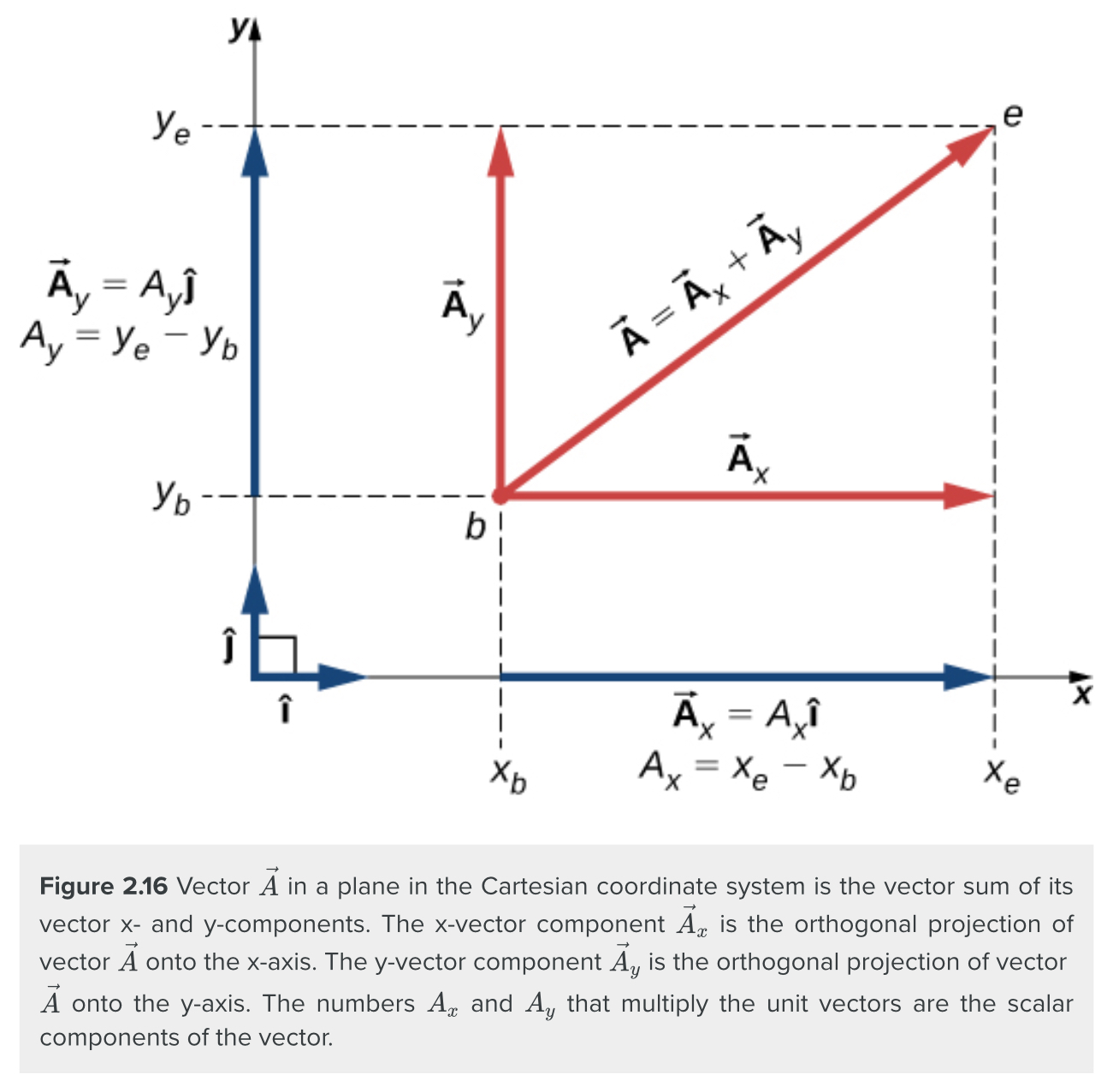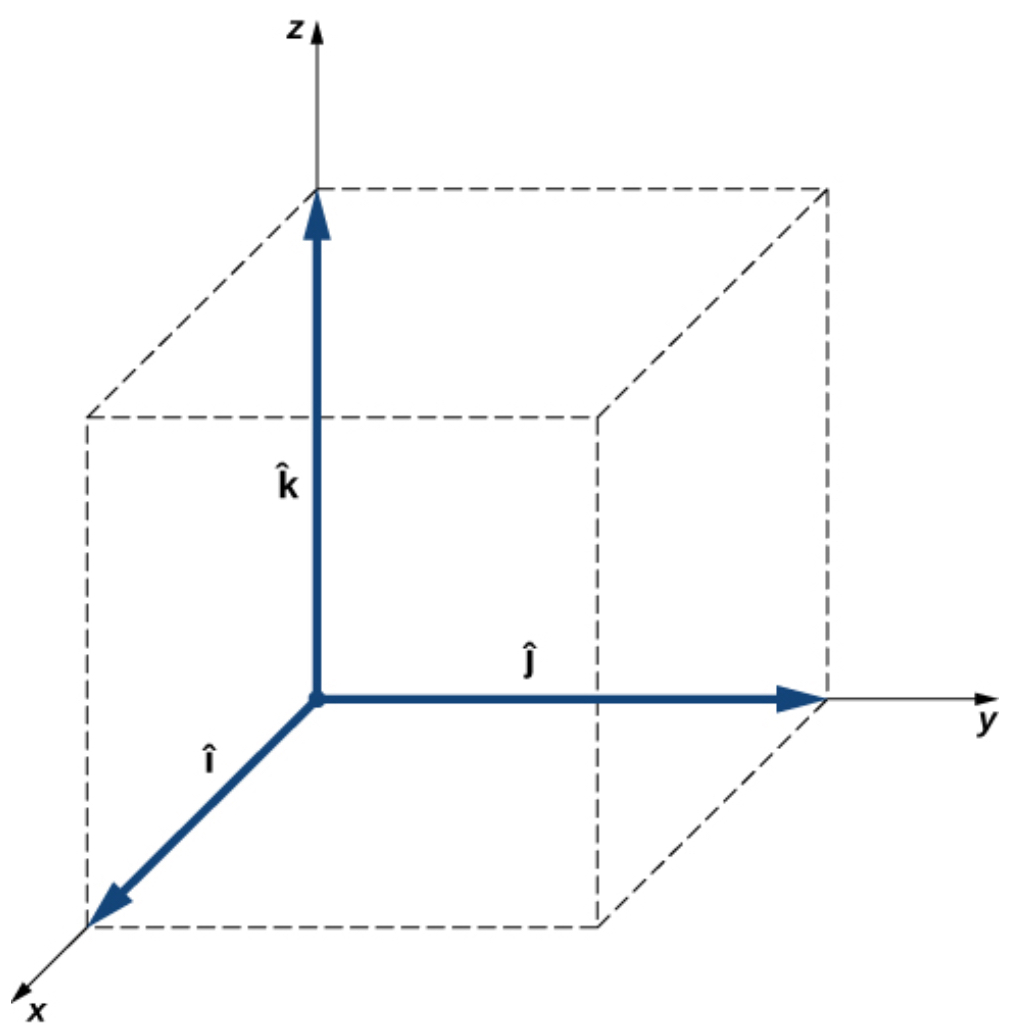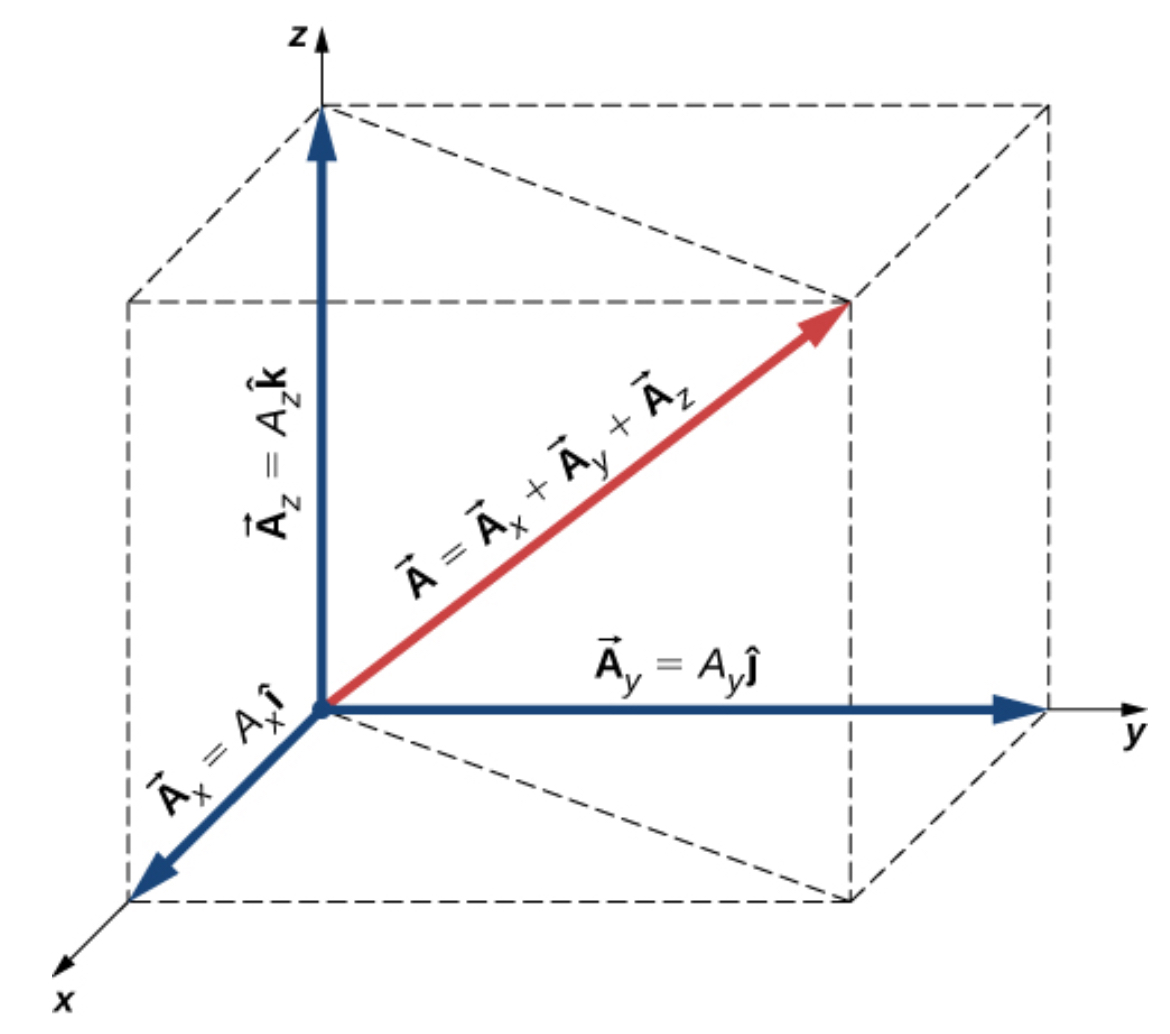Chapter 1: Fundamental Concepts

# 1.2 XYZ Coordinate Frame

We need a standard to be able to share a common language. The Cartesian coordinate frame lets us express the location of a point so that others can understand what we’re talking about.In this section, we’ll look at 2d and 3d coordinate frames.

# 1.2.1 Cartesian Coordinate Frame in 2D

Vectors are usually described in terms of their components in a coordinate system. Even in everyday life we naturally invoke the concept of orthogonal projections in a rectangular coordinate system. For example, if you ask someone for directions to a particular location, you will more likely be told to go 40 km east and 30 km north than 50 km in the direction 37° north of east.

In a rectangular (Cartesian) x-y coordinate system in a plane, a point in a plane is described by a pair of coordinates (xy). In a similar fashion, a vector $\vec A$ in a plane is described by a pair of its vector coordinates. The x-coordinate of vector  is called its x-component and the y-coordinate of vector  is called its y-component. The vector x-component is a vector denoted by $\vec A_x$. The vector y-component is a vector denoted by $\vec A_y$. In the Cartesian system, the x and y vector components of a vector are the orthogonal projections of this vector onto the x– and y-axes, respectively. In this way, following the parallelogram rule for vector addition, each vector on a Cartesian plane can be expressed as the vector sum of its vector components:

$\vec A =\vec A_x+\vec A_y$

As illustrated in the figure below, vector $\vec A$ is the diagonal of the rectangle where the x-component $\vec A_x$ is the side parallel to the x-axis and the y-component $\vec A_y$ is the side parallel to the y-axis. Vector component $\vec A_x$ is orthogonal to vector component $\vec A_y$.It is customary to denote the positive direction on the x-axis by the unit vector i and the positive direction on the y-axis by the unit vector j. Unit vectors of the axes, i and j, define two orthogonal directions in the plane. As shown in the figure above, the x– and y– components of a vector can now be written in terms of the unit vectors of the axes:

$\vec A_x = A_x\underline{\hat{i}}$

$\vec A_y = A_y\underline{\hat{j}}$

The vectors $\vec A_x and \vec A_y$ defined by the figure above are the vector components of vector $\vec A$ . The numbers Ax and Ay that define the vector components above are the scalar components of vector $\vec A$ . Combining the diagram above with the equations above, we obtain the component form of a vector:

$\vec A=A_x\underline{\hat {i}} + A_y\underline{\hat {j}}$

If we know the coordinates b(xb, yb) of the origin point of a vector (where b stands for “beginning”) and the coordinates e(xe, ye) of the end point of a vector (where e stands for “end”), we can obtain the scalar components of a vector simply by subtracting the origin point coordinates from the end point coordinates:

$A_x = x_e - x_b$

$A_y = y_e - y_b$

# 1.2.2. Cartesian Coordinate Frame in 3D

To specify the location of a point in space, we need three coordinates (xyz), where coordinates x and y specify locations in a plane, and coordinate z gives a vertical position above or below the plane. Three-dimensional space has three orthogonal directions, so we need not two but three unit vectors to define a three-dimensional coordinate system. In the Cartesian coordinate system, the first two unit vectors are the unit vector of the x-axis i and the unit vector of the y-axis j. The third unit vector k is the direction of the z-axis, as can be seen below. The order in which the axes are labeled, which is the order in which the three unit vectors appear, is important because it defines the orientation of the coordinate system. The order xyz, which is equivalent to the order , defines the standard right-handed coordinate system (positive orientation).In three-dimensional space, vector $\vec A$  has three vector components: the x-component $\vec A_x = A_x\underline{\hat{i}}$ , which is the part of vector$\vec A$ along the x-axis; the y-component $\vec A_y = A_y\underline{\hat{j}}$ , which is the part of $\vec A$  along the y-axis; and the z-component $\vec A_z = A_z \underline{\hat{k}}$, which is the part of the vector along the z-axis. A vector in three-dimensional space is the vector sum of its three vector components:

$\vec A= A_x\underline{\hat{i}}+A_y\underline{\hat{j}}+A_z\underline{\hat{k}}$

If we know the coordinates of its origin b(xb, yb, zb) and of its end e(xe, ye, ze) its scalar components are obtained by taking their differences, and the z-component is given by:

$A_z=z_e-z_b$

Magnitude A is obtained by the following equation:

$A=\sqrt {A_{x}^{2}+A_{y}^{2}+A_{z}^{2}}$

This expression for the vector magnitude comes from applying the Pythagorean theorem twice. As seen in the figure below, the diagonal in the x-y plane has length $\sqrt{A_{x}^{2}+A_{y}^{2}}$  and its square adds to the square Az2 to give A2 . Note that when the z-component is zero, the vector lies entirely in the x-y plane and its description is reduced to two dimensions.Source: University Physics Volume 1, OpenStax CNX, https://courses.lumenlearning.com/suny-osuniversityphysics/chapter/2-2-coordinate-systems-and-components-of-a-vector/

The Cartesian coordinate frame is a right-orthogonal system. This will matter when we start looking at the right-hand rule in section 3.1. What is means is that when you draw two of the directions (say x and y), then z must go either up or down. We’ll get into that more, later.

Key Takeaways

Basically: The Cartesian coordinate frame gives us a way to communicate the location of a point in space. In 2-d we use [x, y] and in 3-d we also include z: [x, y, z].

Application: If I am trying to walk across the room, I can walk in a straight line for 5 steps, or I can take 3 steps to the right and 4 steps ahead. If I wanted to describe the position of where I went in the Cartesian coordinate frame, it would be [3, 4] assuming x is to the right and y is straight ahead.

Looking ahead: This will connect with everything we do in this class, especially with cross-products (1.5), torque (1.6), and equilibrium equations (everywhere).